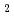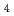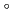Densities of Bunsen reaction solutions in the iodine-sulfur process were measured with an oscillating U-tube density meter. Two types of the solutions were prepared to simulate sulfuric acid solutions and hydriodic acid solutions of the Bunsen reaction step. The former solution ranged in concentration from 0 to 45 wt% of sulfuric acid containing HI and Iof 0-2 mole%; the latter solution contained 0-17 mole% I, 1-15 mole% HI and 0-2 mole% HSO. The temperature of the measured solution were 10-60C. It was found that, in both solutions, the effect of HI and Iconcentration on the density could well be represented by using a kind of mole fraction of iodine atom. Based on the finding, a set of correlation equations between the densities and the compositions were derived. Additionally, viscosities of ploy-hydriodic acid were measured using an oscillating viscosity meter in temperature range of 5-40C, and in the composition range of 0-17 mole% Iand 1-15 mole% HI; a empirical equation to calculate viscosity from the composition and the temperature are obtained.